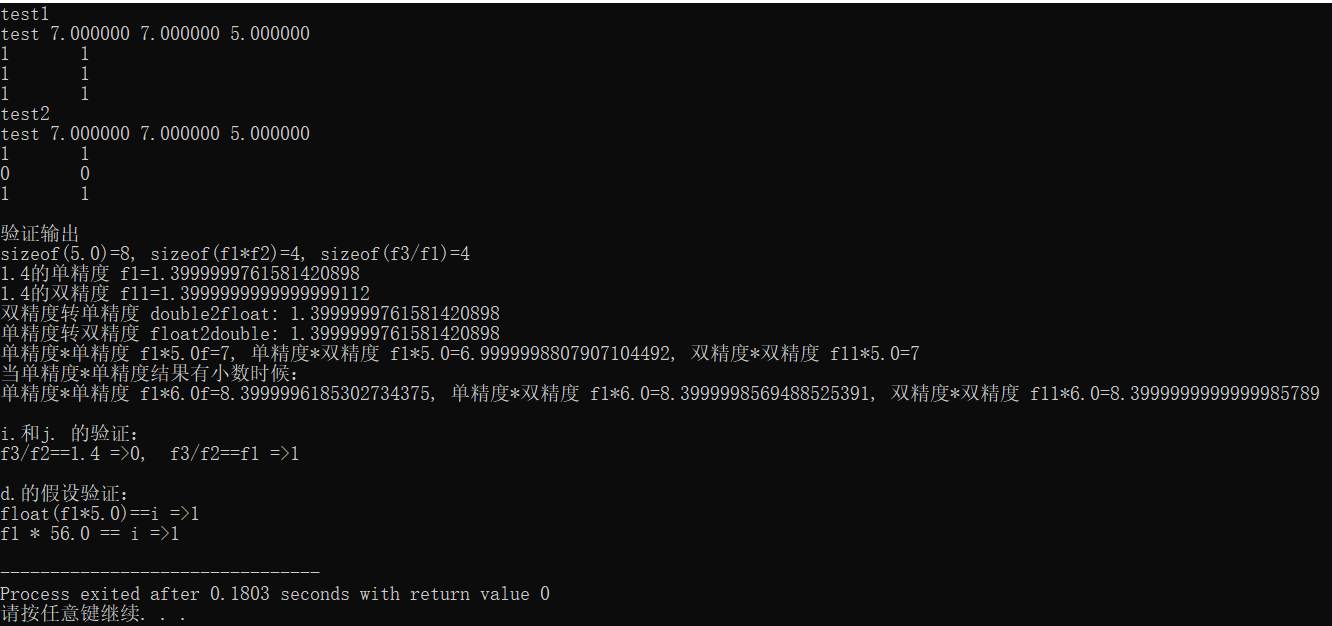2021-02-09 14:42

# 一个关于数据类型的问题

``````#include <iostream>
using namespace std;
int main(void)
{
float f1 = 1.4;
float f2 = 5.0;
float f3 = 7.0;
int i = 7;
printf("test %f %f %f\n", f1 * f2, f1 * 5.0, f3 / f1);
cout << (f1 * f2 == i) << '\t' << (f1 * f2 == f3) << endl;
cout << (f1 * 5.0f == i) << '\t' << (f1 * f2 == f1 * 5.0f) << endl;
cout << (f3 / f1 == 5.0) << '\t' << (f3 / f1 == f2);
return 0;
}``````
``````#include <iostream>
using namespace std;
int main(void)
{
float f1 = 1.4;
float f2 = 5.0;
float f3 = 7.0;
int i = 7;
printf("test %f %f %f\n", f1 * f2, f1 * 5.0, f3 / f1);
cout << (f1 * f2 == i) << '\t' << (f1 * f2 == f3) << endl;
cout << (f1 * 5.0 == i) << '\t' << (f1 * f2 == f1 * 5.0) << endl;
cout << (f3 / f1 == 5.0) << '\t' << (f3 / f1 == f2);
return 0;
}``````

• 写回答
• 好问题 提建议
• 关注问题
• 收藏
• 邀请回答

#### 7条回答默认 最新

•Briwisdom 2021-02-15 00:43
已采纳

这两段代码涉及的是float类型和double类型数据在计算机中的存储问题。以下是个人理解，仅供参考吧。

用到的基础知识：

1， C++中默认5.0是一个double类型，5.0f表示指定这是一个float类型的数字。

2， 精度（float*double)=> double*double=精度（double), 精度(float*float)=精度(float), 精度(double*double)=精度(double)

3,  float类型用4个字节表示，double类型用8个字节表示，double可以用更多的位表示小数点部分（这部分知识可以参考数字在计算机中的表示，https://blog.csdn.net/ultrakang/article/details/39322275），所以有小数的情况下，double比float精度更高。而float转double精度不变，double转float精度降低。

4，float和double转换中精度降低的情况只作用在存在小数位情况。且只存在于小数位的非0尾数大于23bit情况下（因为float尾数是23个bit, double尾数是52个bit)。

再来看本例中给定的数据 f1=1.4; f2=5.0; f3=7.0; i=7。共进行了如下的运算结果比对，跟着对应的结果分析。

a.  f1 * f2 == i       =》1，左边 f1*f2 等效于 float*float，结果仍然是float，恰好是整数，所以f1 * f2 == i 成立。

b.  f1 * f2 == f3     =》1，左边 同 a. ，右边f3是float类型，不涉及数据类型转换，精度不变，所以f1 * f2 == f3 成立。

c.  f1 * 5.0f == i    =》1，左边 f1*5.0f 等效于float*float，其它同a. ，所以f1 * 5.0f == i  成立 。

d.  f1 * 5.0 == i    =》0，左边 f1*5.0等效于double*double, f1=1.4，1.4作为float和double两种类型在计算机存储的精度是不同的，并且结果也是double类型，精度更高，所以f1 * 5.0 == i 不成立，如果将f1*5.0转换成float类型则成立，即：float(f1*5.0)==i 成立。或者将本例中f1=1.4换成f1=0.125（即一个小数点位数可在23bit之内表示的数 , 则f1 * 56.0 == i 成立，因为0.125值的float和double类型在计算机存储中是一样的。

e.  f1 * f2 == f1 * 5.0f   =》1，右边f1 * 5.0f是float*float，不涉及类型转换问题，同b. 所以f1 * f2 == f1 * 5.0f 成立。

f.   f1 * f2 == f1 * 5.0   =》0，右边同d. 1.4在计算机中作为float和double表示的精度不同，f1*5.0是double*double=double，比f1*f2的float*float=float精度更高，所以f1 * f2 == f1 * 5.0不成立。

g.  f3 / f1 == 5.0     =》1,  左边float/foat=float，结果用float类型表示，小数位尾数为0，右边double类型的5.0的小数位尾数也都是0，所以f3 / f1 == 5.0 成立。可以结合下面的 i. 来理解。

h.  f3 / f1 == f2      =》1， 左边和右边都是float类型，精度不变，所以 f3 / f1 == f2成立。可以结合下面的j. 来理解。

补充：

i.  f3 / f2 == 1.4   =》0, 左边结果float类型，右边1.4是double类型，精度不同，所以f3 / f2 == 1.4不成立。

j. f3 / f2 == f1   =》1，右边类型是float，1.4用float表示，不涉及精度降低问题，所以f3 / f2 == f1 成立。

cout默认打印出7位有效数字，所以第一行打印的结果都一样。

上面的验证结果如图（代码附下）：代码：

``````#include <iostream>

float f1 =1.4;
float f2 = 5.0;
float f3 = 7.0;
int i = 7;
using namespace std;

void test1()
{
printf("test %f %f %f\n", f1 * f2, f1 * 5.0, f3 / f1);
cout << (f1 * f2 == i) << '\t' << (f1 * f2 == f3) << endl;
cout << (f1 * 5.0f == f3) << '\t' << (f1 * f2 == f1 * 5.0f) << endl;
cout << (f3 / f1 == 5.0) << '\t' << (f3 / f1 == f2);
}

void test2()
{
printf("test %f %f %f\n", f1 * f2, f1 * 5.0, f3 / f1);
cout << (f1 * f2 == i) << '\t' << (f1 * f2 == f3) << endl;
cout << (f1 * 5.0 == f3) << '\t' << (f1 * f2 == f1 * 5.0) << endl;
cout << (f3 / f1 == 5.0) << '\t' << (f3 / f1 == f2);
}
int main(void)
{
cout<<"test1"<<endl;
test1();
cout<<endl<<"test2"<<endl;
test2();

cout<<endl<<endl<<"验证输出"<<endl;
double f11=1.4;
cout<<"sizeof(5.0)="<<sizeof(5.0)<<", sizeof(f1*f2)=" <<sizeof(f1*f2)<<", sizeof(f3/f1)=" <<sizeof(f3/f1)<<endl;
cout.precision(20);
cout<<"1.4的单精度 f1="<<f1<<endl<<"1.4的双精度 f11="<<f11<<endl;
cout<<"双精度转单精度 double2float: "<<float(f11)<<endl;
cout<<"单精度转双精度 float2double: "<<double(f1)<<endl;

cout<<"单精度*单精度 f1*5.0f="<<f1*5.0f<<", 单精度*双精度 f1*5.0="<<f1*5.0<<", 双精度*双精度 f11*5.0="<<f11*5.0<<endl;
cout<<"当单精度*单精度结果有小数时候："<<endl;
cout<<"单精度*单精度 f1*6.0f="<<f1*6.0f<<", 单精度*双精度 f1*6.0="<<f1*6.0<<", 双精度*双精度 f11*6.0="<<f11*6.0<<endl;

cout<<endl<<"i.和j. 的验证："<<endl;
cout<<"f3/f2==1.4 =>"<< (f3/f2==1.4)<<",  f3/f2==f1 =>"<<(f3/f2==f1)<<endl;

cout<<endl<<"d.的假设验证："<<endl;
cout<<"float(f1*5.0)==i =>"<<(float(f1*5.0)==i)<<endl;
f1=0.125;
cout<<"f1 * 56.0 == i =>"<< (f1 * 56.0 == i)<<endl;
return 0;

}``````

已采纳该答案
评论
解决 1 无用
打赏 举报
•歇歇 2021-02-09 15:01

你运行一下就知道了

评论
解决 无用
打赏 举报
•书山客 2021-02-09 15:04

第一个：

test 7.000000 7.000000 5.000000
1    1
1    1
1    1

第二个：

test 7.000000 7.000000 5.000000
1    1
0    0
1    1

C语言中默认的浮点型常量类型为double类型，所以直接写5.0，程序执行时会存在一个将双精度浮点型变量转化为单精度浮点型变量的过程

评论
解决 无用
打赏 举报
•m0_51771971 2021-02-09 15:05

楼主知道结果但是不知道为什么

本题的重点在于为什么有些输出为1而另外一些输出为0，以及原因是什么

评论
解决 无用
打赏 举报
•qt码农C 2021-02-09 16:43

这种现象应该是C++的bug，没有必要弄清楚，记得这种特殊情况就可以了

评论
解决 无用
打赏 举报
•书山客 2021-02-11 13:33

是数据转换的问题，详细的可以搜索看下double和float两种类型数据结构存储占位相关内容，具体的一两句话也解释不清楚

评论
解决 无用
打赏 举报
•niushaolongniuniu 2021-02-13 18:32

f1 * 5.0编译器将其转化为double类型，i转换为float类型，所以不相等

评论
解决 无用
打赏 举报